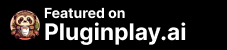# FormulaChatGPT

Sale price\$0.00## FormulaChatGPT AI app

### Fast formula gen & error fix in spreadsheets.

Why Install FormulaChatGPT AI to replace a human task?
Data and Analytics Task and Project Management Utilities and Tools

## AI Information

### What is FormulaChatGPT

FormulaChatGPT Plugin: FormulaChatGPT is a variable that represents an unknown value in a mathematical equation or formula. It can be used in a wide range of applications, from basic arithmetic calculations to complex data analysis. In spreadsheet programs like Excel and Google Sheets, FormulaChatGPT can be used in formulas to perform calculations based on user-defined inputs. For example, if you want to calculate the total cost of a product based on its price and quantity, you can use the formula =price*quantity, where price and quantity are the user-defined inputs and FormulaChatGPT represents the total cost. The FormulaChatGPT Plugin can help you generate accurate and reliable formulas that use FormulaChatGPT and other variables to streamline your spreadsheet workflows and improve data analysis precision.

## TLDR: AI for Fast formula gen & error fix in spreadsheets. Copy and paste these prompts into FormulaChatGPT.

### FormulaChatGPT Prompts

#### Pluginplay prompts for FormulaChatGPT### FormulaChatGPT can be installed on

FormulaChatGPT - Opensource ChatGPT Plugin

### Who is FormulaChatGPT for?

2. Students who need to organize and analyze data for research projects.
3. Small business owners who use spreadsheets for inventory management and sales tracking.
4. Data analysts who need to manipulate and analyze large datasets.
5. Freelancers who use spreadsheets for project management and time tracking.

Install FormulaChatGPT on ChatGPT Plugin Store

### What are the use cases for FormulaChatGPT?

The AI Google Sheets and Excel Formula Bot Generator by FormulaChatGPT can be used in a variety of business and personal use cases. Here are some potential use cases:

1. Financial Analysis: The tool can be used to generate complex financial formulas, such as calculating compound interest, analyzing cash flow, and forecasting revenue.

2. Sales and Marketing: The tool can be used to generate formulas for analyzing sales data, calculating conversion rates, and forecasting sales trends.

3. Human Resources: The tool can be used to generate formulas for tracking employee performance, calculating payroll, and analyzing employee turnover rates.

4. Project Management: The tool can be used to generate formulas for tracking project progress, calculating project costs, and analyzing project risks.

5. Education: The tool can be used by educators to generate formulas for grading assignments, calculating student averages, and analyzing student performance.

6. Personal Finance: The tool can be used by individuals to generate formulas for tracking personal expenses, calculating savings goals, and analyzing investment